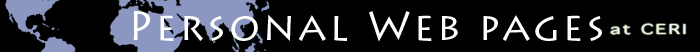Earth structure EarthParam  — returns Earth's physical parameters for a given depth profile (Matlab). MinVel  — calculates mineral and aggregate elastic parameters at given pressures and temperatures (Matlab). TomoSVD  — solves 3-D tomographic problems with singular value decomposition (Matlab and C). Seismology FDWave  — calculates 2-D and 3-D finite difference wavefields for fully anisotropic media (Matlab). 8-26-08 Minor efficiency improvements, added anelasticity and ability to solve 1-D problems. bb  — beach-ball diagram of earthquake focal mechanism(s) (Matlab). 3-28-08 Input can now either be strike dip and rake or the six independent moment tensors Seismic Hazard USGS Hazard Codes  — official USGS hazard codes used for the 1996 national seismic hazard maps (Fortran90 for SunOS). OpenSHA  — an interactive application allowing the user to evaluate various elements of probabilistic seismic hazard assessment (Java). General utilities Shift  — shifts 1-D series (Matlab). Gauss  — generates gaussian series (Matlab). Gauss2  — generates a gaussian surface (Matlab). FilterG  — filters series by convolution with a gaussian (Matlab). CERI Home